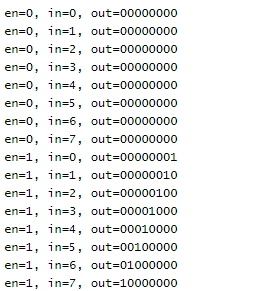# Verilog Code of Decoder | 3 to 8 Decoder Verilog Code

### 3 to 8 Decoder Verilog Code

#### 3 to 8 decoder Verilog Code using case statement

In this post we are going to share with you the Verilog code of decoder. As you know, a decoder asserts its output line based on the input. For a 3 : 8 decoder, total number of input  lines is 3 and total  number of output lines is 8. Based on the input, only one output line will be at logic high.

The Verilog code for 3:8 decoder with enable logic is given below.

### 3:8 Decoder Verilog Code

```module decoder3_to_8( in,out, en);
input [2:0]  in;
input en;
output [7:0] out;
reg [7:0] out;

always @( in or en)
begin

if (en)
begin
out=8'd0;
case (in)
3'b000: out=1'b1;
3'b001: out=1'b1;
3'b010: out=1'b1;
3'b011: out=1'b1;
3'b100: out=1'b1;
3'b101: out=1'b1;
3'b110: out=1'b1;
3'b111: out=1'b1;
default: out=8'd0;
endcase
end
else
out=8'd0;
end
endmodule
```

The Test bench for 3:8 Decoder is given below.

### 3:8 Decoder Test Bench

```module decoder_tb;
wire [7:0] out;
reg en;
reg [2:0] in;
integer i;

decoder3_to_8 dut(in,out,en);

initial begin
\$monitor( "en=%b, in=%d, out=%b ", en, in, out);
for ( i=0; i<16; i=i+1)
begin
{en,in}  = i;
#1;
end
end
endmodule
```

### The output is is given below.If you have any query/suggestion please feel free to comment below the post.View all posts by admin →
Article Rating
Subscribe
Notify ofInline FeedbacksNithin
2 years ago

Is there any alternative codeSin
2 years ago